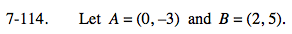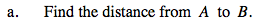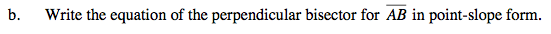### Home > PC > Chapter 7 > Lesson 7.3.2 > Problem7-114

7-114.Make a sketch of the points and use the Pythagorean Theorem to find the distance between the points.You need a point (midpoint) and the slope of the perpendicular line so that you can use the point-slope form of a line.

Find the midpoint by:

Perpendicular slope would be negative the reciprocal of the slope between A and B.